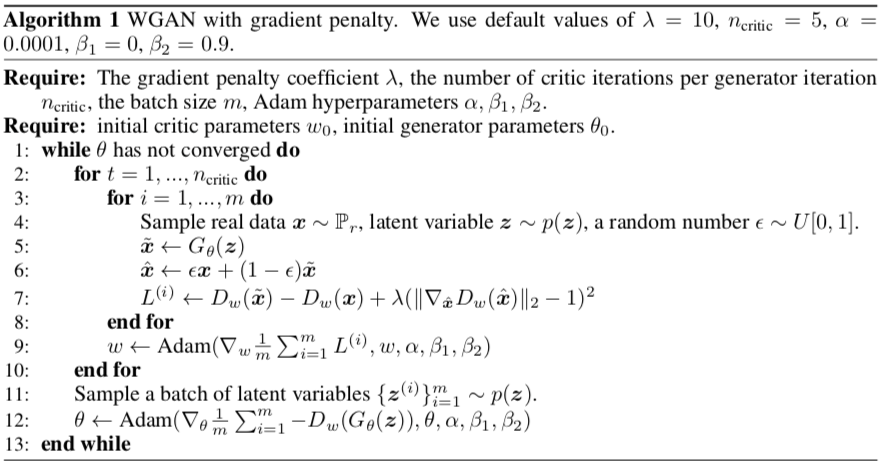Improved Training of Wasserstein GANs@NIPS17.

The paper analyze the reason why Wasserstein GANs might still fail to converge occasionally and present a simple but effective solution to address it.

WGAN requires that the discriminator (critic) to be a 1-Lipschitz function, which the authors enforce through weight clipping. The weight-clipping often results in extremely simple functions, and thus losing the capacity to act as a good critic.

First recall the 1-Wasserstein distance used by WGAN in its Kantorovich-Rubinstein duality form:

$W \left( \mathbb { P } _ { r } , \mathbb { P } _ { \theta } \right) = \sup _ { \| f \| _ { L } \leq 1 } \mathbb { E } _ { x \sim \mathbb { P } _ { r } } [ f ( x ) ] - \mathbb { E } _ { x \sim \mathbb { P } _ { g} } [ f ( x ) ].$

In the original WGAN, the authors propose to clip the weights of critic to lie within a compact space $$[-c, c]$$ to enforce Lipschitz constraint. This may push the weights towards several extreme values, which degrades the expressive power of the critic and results in poor performance.

WGAN with gradient penalty (WGAN-GP), the new loss function for the generator

$L(g_\theta) = \sup _{f}\ \mathbb { E } _ { x \sim \mathbb { P } _ { r } } [ f ( x ) ] - \mathbb { E } _ { x \sim \mathbb { P } _ { g} } [ f ( x ) ] - \lambda \mathbb{E}_{x \sim \mathbb{P}_m} [(\|\nabla_x f(x)\|_2 - 1)^2],$

where $$\mathbb{P}_m$$ is a uniform distribution along straight lines between pairs of points sampled from the data distribution $$\mathbb{P}_r$$ and the generator distribution $$\mathbb{P}_g$$, i.e., $$\mathbb{P}_m = \epsilon \mathbb{P}_r + (1 - \epsilon) \mathbb{P}_g, \epsilon \sim U[0, 1]$$.

Then we minimize $$L(g_\theta)$$ with respect to $$\theta$$, from which we can see the critic $$f(x)$$ only serves to define the loss function for the generator.